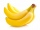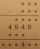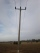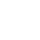# Calculator subtracting two mixed numbers

The calculator performs basic and advanced operations with mixed numbers, fractions, integers, decimals. Mixed fractions are also called mixed numbers. A mixed fraction is a whole number and a proper fraction combined, i.e. one and three-quarters. The calculator evaluates the expression or solves the equation with step-by-step calculation progress information. Solve problems with two or more mixed numbers fractions in one expression.

### 71/2 - 53/4 = 7/4 = 1 3/4 = 1.75

Spelled result in words is one and three quarters (or seven quarters).

### Calculation steps

1. Conversion a mixed number 7 1/2 to a improper fraction: 7 1/2 = 7 1/2 = 7 · 2 + 1/2 = 14 + 1/2 = 15/2

To find new numerator:
a) Multiply the whole number 7 by the denominator 2. Whole number 7 equally 7 * 2/2 = 14/2
b) Add the answer from previous step 14 to the numerator 1. New numerator is 14 + 1 = 15
c) Write a previous answer (new numerator 15) over the denominator 2.

Seven and one half is fifteen halfs
2. Conversion a mixed number 5 3/4 to a improper fraction: 5 3/4 = 5 3/4 = 5 · 4 + 3/4 = 20 + 3/4 = 23/4

To find new numerator:
a) Multiply the whole number 5 by the denominator 4. Whole number 5 equally 5 * 4/4 = 20/4
b) Add the answer from previous step 20 to the numerator 3. New numerator is 20 + 3 = 23
c) Write a previous answer (new numerator 23) over the denominator 4.

Five and three quarters is twenty-three quarters
3. Subtract: 15/2 - 23/4 = 15 · 2/2 · 2 - 23/4 = 30/4 - 23/4 = 30 - 23/4 = 7/4
For adding, subtracting, and comparing fractions, it is suitable to adjust both fractions to a common (equal, identical) denominator. The common denominator you can calculate as the least common multiple of both denominators - LCM(2, 4) = 4. In practice, it is enough to find the common denominator (not necessarily the lowest) by multiplying the denominators: 2 × 4 = 8. In the next intermediate step, the fraction result cannot be further simplified by canceling.
In words - fifteen halfs minus twenty-three quarters = seven quarters.

## Mixed fractions in word problems:

• GavinGavin had 3 gallons of orange juice ready to serve at the breakfast party. His family drank 2 ⅛ gallons. How much is left? Show your work with a numberline, area model or grid.
• Sum of mixedThe sum of two mixed numbers is 15½. One of the number is 5 ¾, what is the other?
• Cups of punchCyka made 6 19/20 cups of punch punch at two different types of juice in it. If the punch had 4 1/5 cups of one type of juice how many cups of the other type of juice did it have?
• Sum of 20Sum of the two numbers is 15 1/6. If one of the numbers is 2 2/3, find the other number.
• DenissaDenissa has 18 9/10 kilos of bananas to sell if she was able to sell 15 ⅗. How many kilos of bananas were left?
• TyronTyron attended his online class 2 hours a day. He already attend 1 3/4 hours, how many more hours will Tyron attend his class?
• Bike pathBill was riding his bike on a bike path that was 5 1/4 miles long. He rode his bike awhile and stopped for s drink or water. Then he rode 2 7/10 miles and was at the end of the path. How far had Bill ridden before he stopped for water?
• Difference mixed fractionsWhat is the difference between 4 2/3 and 3 1/6?
• Light-yearsPlanet X is 10 light-years away from Earth. Planet Y is 8 1/2 light-years away from Earth. How much farther away is Planet X?
• Sum of 18Sum of two fractions is 4 3/7. If one of the fractions is 2 1/5 find the other one .
• Equation with mixed 2A number, X, is subtracted from 8 1/4. The result is 12 3/5. What is the value of X?
• MarcosMarcos wants to buy grass seed to cover his whole lawn, except for the pool. The pool is 7 1/2 m by 3 1/4 m. Find the area the grass seed needs to cover. Dimensions of the lawn are 50 m x 150 m.
• PizzasBilly ate 1 1/4 pizzas and John ate 1 2/3 pizzas. How much more pizza did John eat than Billy?
• The petAnanya has a bunny. She bought 4 7/8 pounds of carrots. She fed her bunny 1 1/4 pounds of carrots the first week. She fed her bunny 5/6 pounds of carrots the second week. All together, how many pounds of carrots did she feed her bunny? 1. Draw a tape diag
• KevinKevin is baking brownies and needs peanuts.  The recipe calls for 1 and 2/3 cups of peanuts.  Kevin only has 5/6 of a cup of peanuts.  How many more cups of peanuts does Kevin need to have the correct amount?
• A burgerThe water starts with 41 1/4 cups of water how much water is left after ten 3 5/8 Scoops of water are removed?
• EvanEvan, Samuel, and Alex are doing a biking relay on the Key Biscayne Bridge. Altogether, they bike a total 7 miles. If Evan bikes 2 1/3 of a mile and Samuel bikes 2 1/4 of a mile, what distance does Alex bike?